# Linear Discriminant Analysis

## Introduction

As technology advances and grows, people are connected, devices are connected. This leads to a massive increase in data and increased requirement of storage spaces. While the data is increasing, data hackers and crackers are creating various ways and algorithms to steal that data.  Reduction of data dimensions was introduced to overcome such fear, and it can indirectly help in the security and privacy of data.Before jumping to linear discriminant analysis, let us first understand Fisher’s linear discriminant.

## What is Fisher’s Linear Discriminant?

Linear Discriminant Analysis is based upon Fisher’s linear discriminant. The FLD is a classification method that focuses on mapping the high-dimensional data points. The projection’s main idea is to maximize the data points between two classes and minimize the variance within the class variables.

Let us understand it with an example:

Suppose there is a dataset with the following points with two classes, red and blue.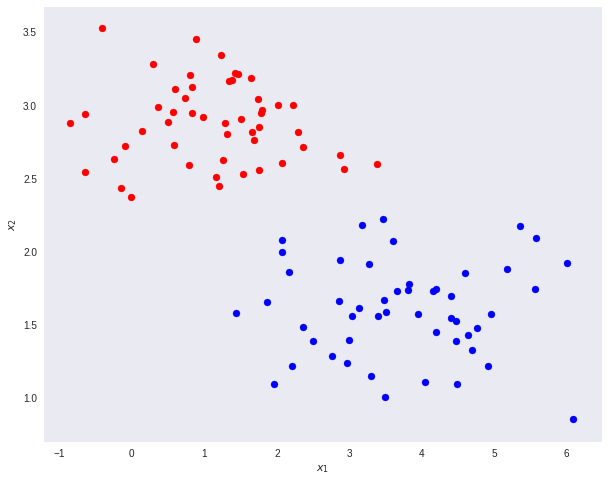Now according to the Fisher’s mathematical equations: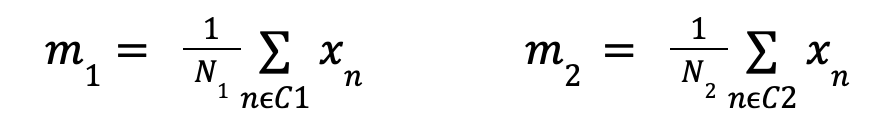Where,

N1 and N2 represent the number of points in classes C1 and C2, respectively.

Projecting the hyperplane will ensure minimum variance and maximum data points between the classes.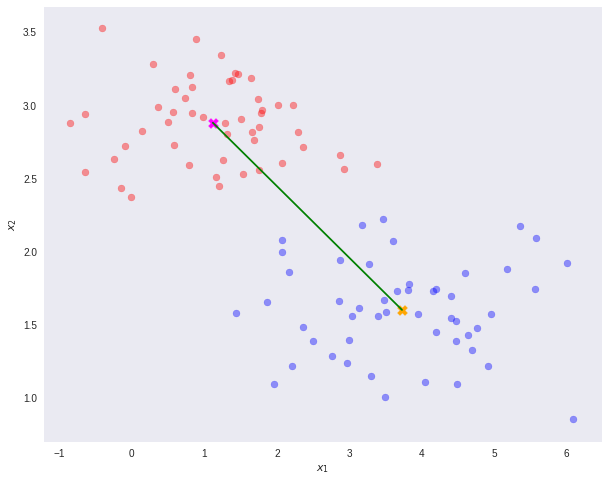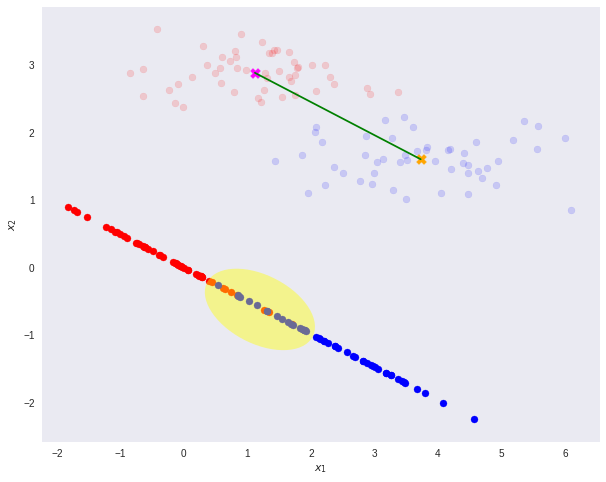In the image, it can be observed that the hyperplane has divided the data points. The red portion is for Class 1, the blue is for Class 2, while the yellow highlighted potion is the overlapped points.

Linear Discriminant Analysis is somewhat related to Fisher's Linear Discriminant theorem; let us understand it.

## What is Linear Discriminant Analysis?

Linear Discriminant Analysis is a subset of dimensionality reduction. This technique is used to decipher two or more classes in supervised learning. It maps the linear data from a higher dimensionality to a lower dimensionality. Linear Discriminant Analysis is responsible for expressing a linear relationship between a dependent variable and other features, similar to regression analysis.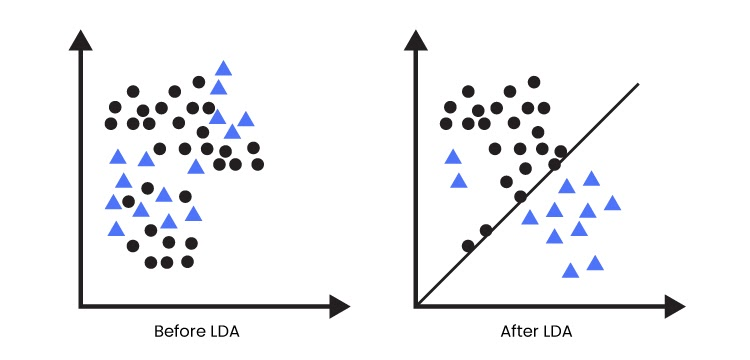## Why Linear Discriminant Analysis?

Although linear discriminant analysis is similar to logistic regression, logistic regression cannot perform multiple classification problems. Therefore, LDA comes into play and solves the problems for multiple classifications with well-oriented classes. It is also used for data pre-processing to reduce the number of features and only model based upon the vital parameters.

## Assumptions of Linear Discriminant Analysis

LDA is sensitive to outliers; therefore, there are some assumptions of discriminant analysis.

• Homoscedasticity: It is a condition where variance is constant.
• Multivariate normality: It is a condition where the variables are normally distributed in a linear combination.
• Multicollinearity: It is a condition where there is a high correlation between the independent variables.
• Independence: The variables are assumed to be independent of each other.

## Limitations of Linear Discriminant Analysis

• It is assumed to be normally distributed
• At times, it is not the best fit for a few categories of variables.

## Applications of Linear Discriminant Analysis

• Face recognition
• Marketing
• Bankruptcy prediction
• Earth Science
• Biomedical studies

Q1. What is the eigenvalue in linear discriminant analysis?
Ans. The eigenvalue in LDA is the root value of the quality of each function. It indicates how the classes differ; the higher the eigenvalue, the better the differentiated functions.

Q2. What is incremental Linear Discriminant Analysis?
Ans. Incremental LDA implements Linear Discriminant Analysis that requires all the samples available in advance. The Feature extraction process is applied by observing the new samples rather than the whole dataset.

Q3. Is Linear Discriminant Analysis a linear model?
Ans. Linear Discriminant Analysis is a linear model just like logistic regression. However, unlike logistic regression, LDA can model on more than two classes.

## Key Takeaways

Linear Discriminant Analysis is a technique of supervised learning where the data points are separated into two or more classes. In this blog, we learned what LDA is, the theory of Fisher's Linear Analysis, the assumptions of LDA, limitations, and applications of LDA. If interested in going deeper, Check out our industry-oriented machine learning course curated by our faculty from Stanford University and Industry experts.